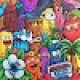# Solve this if you are a genius

Solve this if you are a genius? This quiz showed up on my Facebook news feed.

8 = 56
7 = 42
6 = 30
5 = 20
3 = ?

The pattern looks pretty easy, right?

82— 8 = 56
72— 7 = 42
62— 6 = 30
52— 5 = 20

So what is the correct answer?

Here’s mine:

3 = 3 — even if it’s preceded by a bunch of false statements.

Do you agree?

Update. This is an old post and I thought it had seen all the life it was going to see. Then Angel comes and leaves a comment:

It turns out that the “?” can be whatever we want it to be!

Angel backs up his claim with math. I think we have the definitive answer.

Tags:

<< Next Post
Previous Post >>

## 106 responses to “Solve this if you are a genius”

1.musa

it is interesting my answer is 6 because 8 × 7 = 56
7 × 6 = 42
6 × 5 = 30
5 × 4 = 20
3 × 2 = 6

2.More than seven years later, this problem continues to intrigue people. I’m glad you found it interesting and, ignoring my problem with the equals sign, I agree with you.

Thanks for the visit and comment!

3.Ange

8=56
7=42
6=30
5=20
3=.15

4.Hmm… I’m not sure I know how you got there. Care to expand on your reasoning? :-)

5.Saps

I like the answer of B. Luetkemeyer the most cause if you follow this pattern
8 = 56 7 = 42 (56 â€“ 42 = 14 = 2 Ã— 7) 6 = 30 (42 â€“ 30 = 12 = 2 Ã— 6) 5 = 20 (30 â€“ 20 = 10 = 2 Ã— 5)
then 4 = 15 (20-15 = 5 = 1 Ã— 5)
and 3 = 0 ( 0 Ã— 5)
I think this is a right cause numbers doesn’t really exist like the most things humans created: 3 is not equal to 3 cause 3 isn’t 3, though we might see it as a 3 but it’s just molecules who form something we can see and cause it doesn’t exist and zero doesn’t exist we can say that they are equal for us cause they actually don’t excist so they can’t be so they can’t be equal to eachother but when we have to give this a number then 3 = 0

6.Martin

I also agree with your answer. The way i got it was:
8 * 8 = 64 – 8 = 56
7 * 7 = 49 – 7 = 42
6 * 6 = 36 – 6 = 30
5 * 5 = 25 – 5 = 25
so
3 * 3 = 9 – 3 = 6

Thats how i got my answer.